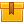# Decimals (A4302)

## Prerequisites

This lesson is designed to build on the skills presented in the first lesson of this program, Whole Number Operations.

## Description

This lesson provides instruction in mathematical operations that require the use of decimals. Participants learn how to read decimal numbers as well as compare their values and round them. Participants also learn how to add, subtract, multiply and divide decimals, both with pencil and paper and with a calculator. The lesson provides many examples of applications at
home and at work.

## Objectives

Use decimals to describe parts of a whole. Read decimal numbers and properly interpret their value. Compare and rank decimal numbers. Round decimals to a specified place value. Properly interpret a target value. Find the upper and lower limits and identify values that fall within the acceptable range. Add decimals using paper and pencil. Use a calculator to add decimals. Use rounding to estimate decimal sums. Use the calculator memory to store subtotals when performing a multi-step addition problem. Subtract decimals using paper and pencil. Use? a calculator to subtract decimals. Use addition to check subtraction, and subtraction to check addition. Properly interpret specifications that have indicated tolerances. Find the upper and lower limits of the specification and identify values that fall within the specified range. Multiply decimals using paper and pencil. Use a calculator to multiply decimals. Properly use zeros as place holders in decimal multiplication. Calculate the area of a rectangular space. Divide decimals using paper and pencil. Use a calculator to divide decimals. Calculate a mean (or average) using sample values that contain decimals. Calculate the range for a sample that contains decimal values.

## ContentThis lesson provides instruction in mathematical operations that require
the use of decimals. Participants learn how to read decimal numbers as
well as compare their values and round them. Participants also learn how
to add, subtract, multiply and divide decimals, both with pencil and
paper and with a calculator. The lesson provides many examples of
applications at home and at work.

•Decimals

0
0 Reviews